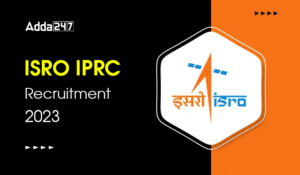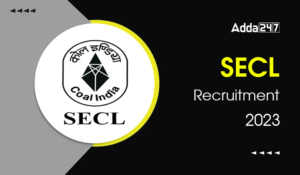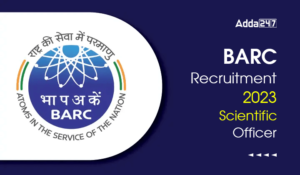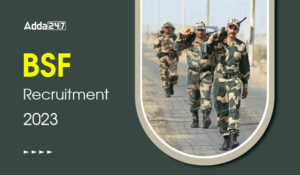Engineering Jobs   »   Mechincal Engineering quizs   »   NHPC JE Recruitment 2021, NHPC JE...

# NHPC JE’21 ME: Daily Practices Quiz. 30-Nov-2021

Quiz: Mechanical Engineering
Exam: NHPC
Topic: Miscellaneous

Each question carries 1 mark
Negative marking: 1/4 mark
Time: 6 Minutes

Q1. Which of the following statements are correct for temperature rise in metal cutting operation?
1. it adversely affects the properties of tool material.
2. It provides in-accuracy during machining.
3. It causes dimensional changes in workpiece and affects accuracy of machining.
4. It can’t distort the accuracy of machine tool itself.
(a) 1 and 2
(b) 2 and 3
(c) 3 and 4 only
(d) 1, 2 and 3

Q2. In an inventory, if there is production and shortage both are involved, then what is the correct formula of EOQ? Where D be the annual demand C_o be the ordering cost, C_h be the holding cost, p be the p be the rate of production, d be the demand or consumption rate and C_b be the shortage cost.
(a) √((2DC_o)/C_h ×p/(p-d)×(C_b+C_h)/C_b )
(b) √((2DC_o)/C_h ×p/(p-d)×(C_b-C_h)/C_b )
(c) √((2DC_o)/C_h ×(p-d)/p×(C_b+C_h)/C_b )
(d) √((2DC_o)/C_h ×p/(p+d)×(C_b+C_h)/C_b )

Q3. What is the reorder level when its lead time is 7 days, consumption rate of a quantity is 20 per day and its safety stock is 50?
(a) 370
(b) 200
(c) 150
(d) 190

Q4. Bolts are designed on the basis of
(a) direct tensile stress
(b) direct shear stress
(c) direct compressive stress
(d) direct bearing stress

Q5. In case of trapezoidal thread, the frictional torque at mean radius while raising load is where,
W = load, d_m = mean diameter, θ= angle of friction, α = Helix angle
(a) (Wd_m)/2×(μsecθ+tanα)/(1-μsecθ.tanα)
(b) (Wd_m)/2×(μsecθ+tanα)/(1+μsecθ.tanα)
(c) (Wd_m)/2×(μsecθ-tanα)/(1-μsecθ.tanα)
(d) (Wd_m)/2×(μsecθ-tanα)/(1+μsecθ.tanα)

Q6. Air at 20°C dry bulb temperature and 40% relative humidity is heated upon 40°C using an electric heater, whose surface temperature is maintained uniformly at 45°C. The efficiency of the heater is
(a) 0.80
(b) 0.20
(c) 0.88
(d) 1

Solutions

S1. Ans. (d)
Sol. The rise of temperature of work piece and tool give rise to
(i) Early wear of tool
(ii) Dimensional in-accuracy of machined surface
(iii) Damage of surface properties of machined component.
So, statement 4 is wrong

S2. Ans. (a)
Sol. If both production and shortage case is considered in an Inventory then the formula of EOQ is
Q^*=√((2DC_o)/C_h ×p/(p-d)×(C_b+C_h)/C_b )
And its total inventory cost (TIC) at EOQ is
〖TIC〗^*=√(2DC_o C_h×p/(p-d)×(C_b+C_h)/C_b )
S3. Ans. (d)
Sol. We know that, ROL=LT×d+SS
Where ROL = Reorder level, LT = Lead time = 7 days, d = consumption rate = 20 and SS = safety stock = 50
ROL=7×20+50=140+50=190

S4. Ans. (a)
Sol. Bolts are designed on the basis of direct tensile stress.

S5. Ans. (a)
T=(Wd_m)/2×(μsecθ+tanα)/(1-μsecθ.tanα)

S6. Ans. (b)
Sol. Given,
t_1=20°C
t_2=40°C
t_3=45°C
BPF=(t_3-t_2)/(t_3-t_1 )=(45-40)/(45-20)=0.2

So, efficiency of the coil is 1-BPF=1-0.2=0.8

Sharing is caring!

•GATE 2023 Admit Card Out, Download Link ...
•TSSPDCL Recruitment 2023 Notification Ou...
•ISRO IPRC Recruitment 2023 Notification ...
•SECL Recruitment 2023 Notification Out, ...
•BARC Recruitment 2023 Scientific Officer...
•Central Railway Recruitment 2023 Apply O...
•BSF Recruitment 2023, Apply Online for J...